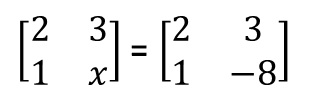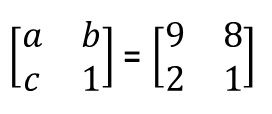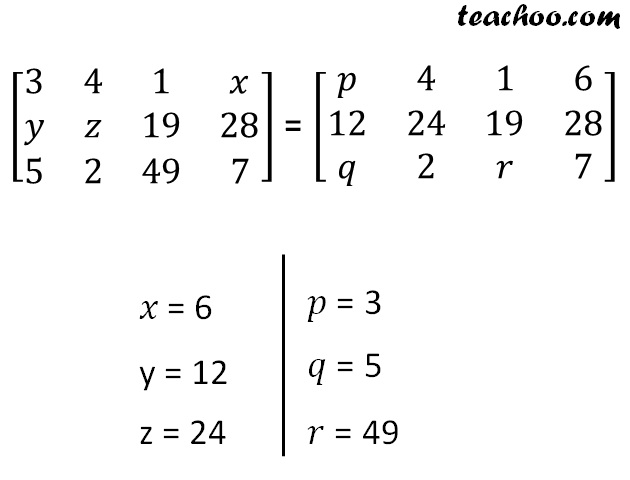If two matrices are equal, then their corresponding elements are equal

ExampleSo, x = −8a = 9,

b = 8

c = 21. Chapter 3 Class 12 Matrices (Term 1)
2. Concept wise
3. Equal matrices

Transcript

[■8(2&3@1&𝑥)] = [■8(2&3@1&−8)] [■8(𝑎&𝑏@𝑐&1)] = [■8(9&8@2&1)] [■8(3&4&1&𝑥@𝑦&𝑧&19&28@5&2&49&7)] = [■8(𝑝&4&1&6@12&24&19&28@𝑞&2&𝑟&7)] 𝑥 = 6 y = 12 z = 24 𝑝 = 3 𝑞 = 5 𝑟 = 49